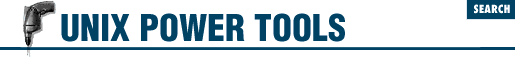home | O'Reilly's CD bookshelfs | FreeBSD | Linux | Cisco | Cisco Exam

#Chapter 49Working with Numbers## 49.5 Base Conversion Using cvtbase

 cvtbase The bc command can be used to convert between different bases ( 49.2 ) , but an easier tool to use is cvtbase . cvtbase is designed specifically to convert from one format to another. For example, to convert a decimal IP address into its hexadecimal equivalent:

```% ```

cvtbase d h
```

```

140.186.65.25
```

8c.ba.41.19```

The first argument, ``` d``` , means that our input will be in decimal form. The second argument, ``` h``` , means that we want the input converted into hexadecimal. In general, the syntax for calling cvtbase is:

```cvtbase ```

input_base output_base
```
```

where input_base and output_base are any of:

``` d, D```

Decimal; i.e., digits ``` 0``` through ``` 9``` .

``` x, h```

Hexadecimal (using lowercase); i.e., ``` 0``` through ``` 9``` and ``` a``` through ``` f``` .

``` X, H```

Hexadecimal (using uppercase); i.e., ``` 0``` through ``` 9``` and ``` A``` through ``` F``` .

``` o, O```

Octal; i.e., digits ``` 0``` through ``` 7``` .

``` b, B```

Binary; i.e., digits ``` 0``` and ``` 1``` .

Any input characters that aren't in the specified set are sent through unchanged. In the example above, the dots (``` .``` ) in the IP address are retained in the hexadecimal output.

- LM49.4 bc's Sine and Cosine Are in Radians49.6 Quick Arithmetic with expr